HLIBpro  2.3.1
Matrix Factorisation

# Unsymmetric Matrices

For unsymmetric matrices, 𝓗𝖫𝖨𝖡𝗉𝗋𝗈 implements LU and LDU factorisation, i.e. the matrix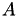is factorised into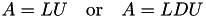with a lower triangular, unit diagonal matrix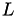, a upper triangular matrix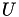and, in the case of LDU a diagonal matrix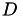(in which caseis also unit diagonal).

Both matrix factorisation are available in two modes:

• block-wise: the matrix factorisation is performed up to leaf matrices in the matrix hierarchy, e.g. dense diagonal matrices are not factorised but inverted.
• point-wise: a real LU (LDU) factorisation is computed, i.e. also dense diagonal blocks are factorised.

In the case of point-wise factorisation, the possibility of a break-down is high because pivoting is only possible to a very small degree for 𝓗-matrices and therefore not implemented in 𝓗𝖫𝖨𝖡𝗉𝗋𝗈. Therefore, block-wise factorisation is the default factorisation mode, since it can only break down in the case of singular diagonal blocks (in which case some remedy is avaibable, see below).

The factorisation methods are implemented in the classes TLU and TLDU. Factorisingusing default parameters can be performed as

TLU fac;
TTruncAcc acc( 1e-4 );
fac.factorise( A, acc );

Here, a block-wise accuracy of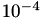were used during LU. By replacing TLU with TLDU, the corresponding factorisation method is used. Furthermore, for both variants, functional forms are available, called lu and ldu:

TTruncAcc acc( 1e-4 );
ldu( A, acc );

## LU-Factor Evaluation: Linear Operators

Matrix factorisation is performed in place, i.e. the individual factors are stored within, thereby overwriting the original content. That also means, thatcan no longer be used as a standard matrix object, e.g. for matrix vector multiplication, since for that, the content needs special interpretation, e.g. first multiply with upper triangular part, then with lower triangular.

Special classes are avaiable in 𝓗𝖫𝖨𝖡𝗉𝗋𝗈 to perform such interpretation, namely:

• TLUMatrix, TLDUMatrix: compute matrix vector product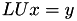and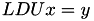respectively,
• TLUInvMatrix, TLDUInvMatrix: compute matrix vector product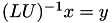and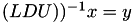respectively.

Since the corresponding objects do not provide standard matrix functionality, they are representatives of the linear operator class TLinearOperator. Linear operators only provide evaluation of the matrix-vector product, which itself is a limited version of the standard TMatrix matrix-vector multiplication since only updates of the destination vector are possible, e.g. either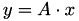or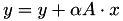However, also the transposed or hermitian evaluation are supported.

TLUMatrix (TLDUMatrix) and TLUInvMatrix (TLDUInvMatrix) objects may be created explicitely or by using the corresponding functions of TLU (TLDU):

auto A_fac = fac.eval_matrix( A ); // return TLUMatrix/TLDUMatrix
auto A_inv = fac.inv_matrix( A ); // return TLUInvMatrix/TLDUInvMatrix
unique_ptr< TVector > x = ... // set up some vector
unique_ptr< TVector > y = ... // set up some vector
// eval A x = y
A_fac->apply( x.get(), y.get(), MATOP_NORM );
// eval A^-1 x = y
A_inv->apply( x.get(), y.get(), MATOP_TRANS );
Remarks
Since the "*Inv*" classes provide evaluation of the inverse, they can be used for preconditioning while solving equation systems iteratively (see ???).

## Factorisation Parameters

So far, matrix factorisation was performed with default parameters. Options for matrix factorisation are provided in the form of a fac_options_t object. With it, you may select point-wise factorisation, e.g.:

fac_options_t facopt;
facopt.eval = point_wise;
TLU fac( facopt );

Also, a progress meter may be assigned for the factorisation:

TConsoleProgressBar progress( cout );
facopt.progress = & progress;

Furthermore, special modifications in case of a factorisation failure, e.g. for a singular matrix or for a matrix with a bad condition, may be activated.

• add_identity: replaceby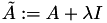, with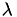being chosen in some interval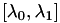until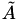can be factorised,
• add_random: replaceby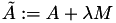, with a random matrix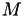andas in the previous case,
• add_random_lr: replaceby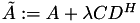, with a random low-rank matrices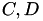with increasing rank until a successfull factorisation can be computed (as before),
• fix_cond: ifhas a very high condition, its singular values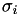will be shifted by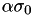, e.g. the largest singular value.

The first three modification techniques are exclusive, i.e. may not be combined, whereas the last method can be used parallel to the others. Furthermore, all modifications only apply to dense diagonal blocks, thereby bounding the computation complexity of all methods such that the overhead can be neglected.

facopt.fix_cond = true;

In most applications, using add_identity (combined with fix_cond) is best for handling errors during factorisation. However, such modifications will usually decrease accuracy and the need to apply them signals an intrinsic problem with the original matrix!

Remarks
In the default case of block-wise factorisation, diagonal blocks will be inverted. If that fails, first the pseudo-inverse is computed and only if that also failes the above modifications will be applied.

# Symmetric/Hermitian Matrices

Ifis symmetric (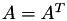) or hermitian (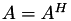), 𝓗𝖫𝖨𝖡𝗉𝗋𝗈 provides the Cholesky (LL) and the LDL factorisation. These are implemented in the classes TLL and TLDL:

TLDL fac;
TTruncAcc acc( 1e-4 );
fac.factorise( A, acc );

or available in functional form:

TTruncAcc acc( 1e-4 );
ll( A, acc );
Remarks
Please note, that TLU and TLDU will not work for symmetric/hermitian matrices as only the lower triangular part is stored and therefore, will throw an exception if called with such a matrix.

Evaluation of a factorisedis provided by TLLMatrix and TLDLMatrix, whereas evaluation of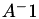is implemented in TLLInvMatrix and TLDLInvMatrix. Please note, that the matrix format, e.g. whether symmetric or hermitian, is lost during factorisation but crucial for matrix evaluation and therefore, has to be provided while constructing these matrix objects:

TLDL fac;
TTruncAcc acc( 1e-4 );
const matform_t matform = A->form()
fac.factorise( A, acc );
auto A_inv = fac.inv_matrix( A, matform );

TLDL uses block-wise factorisation but can be switched to point-wise mode using fac_options_t objects as described above. Also, factorisation modifications/stabilisations may be activated for LDL factorisation. Neither of these is supported for Cholesky factorisation as this always used point-wise mode (block-wise is not possible). It is therefore not as stable as LDL factorisation.

# Simplified Factorisation

Instead of chosing the factorisation technique by yourself and handling the creation of the corresponding linear operators, this can be simplified by using the functions factorise and factorise_inv:

TTruncAcc fac_acc( 1e-4 );
auto A_copy = A->copy;
auto A_inv = factorise_inv( A_copy.get(), acc );

This works for unsymmetric and symmetric/hermitian matrices. Furthermore, options to the factorisation may be provided as an additional argument.